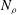Home

# Quasi-geostrophic approximation of anelastic convection

## Abstract

The onset of convection in a rotating cylindrical annulus with parallel ends filled with a compressible fluid is studied in the anelastic approximation. Thermal Rossby waves propagating in the azimuthal direction are found as solutions. The analogy to the case of Boussinesq convection in the presence of conical end surfaces of the annular region is emphasised. As in the latter case, the results can be applied as an approximation for the description of the onset of anelastic convection in rotating spherical fluid shells. Reasonable agreement with three-dimensional numerical results published by Jones, Kuzanyan & Mitchell (J. Fluid Mech., vol. 634, 2009, pp. 291–319) for the latter problem is found. As in those results, the location of the onset of convection shifts outwards from the tangent cylinder with increasing number$\def \xmlpi #1{}\def \mathsfbi #1{\boldsymbol {\mathsf {#1}}}\let \le =\leqslant \let \leq =\leqslant \let \ge =\geqslant \let \geq =\geqslant \def \Pr {\mathit {Pr}}\def \Fr {\mathit {Fr}}\def \Rey {\mathit {Re}}N_{\rho }$ of density scale heights until it reaches the equatorial boundary. A new result is that at a much higher number$N_{\rho }$ the onset location returns to the interior of the fluid shell.

## References

Hide All
MathJax
MathJax is a JavaScript display engine for mathematics. For more information see http://www.mathjax.org.

# Quasi-geostrophic approximation of anelastic convection

## Metrics

### Full text viewsFull text views reflects the number of PDF downloads, PDFs sent to Google Drive, Dropbox and Kindle and HTML full text views.

Total number of HTML views: 0
Total number of PDF views: 0 *Loading metrics...

### Abstract viewsAbstract views reflect the number of visits to the article landing page.

Total abstract views: 0 *Loading metrics...

* Views captured on Cambridge Core between <date>. This data will be updated every 24 hours.

Usage data cannot currently be displayed